# K Nearest Neighbors

#### 1/20/2020

# load python
library(reticulate)
use_python('C:/Users/Andrew/Anaconda3/')
library(knitr)
# load packages
import pandas as pd
import numpy as np
import matplotlib.pyplot as plt
import time
import plotly.express as px
import seaborn as sns

from sklearn.neighbors import KNeighborsClassifier
from sklearn.metrics import confusion_matrix, classification_report, accuracy_score
from prettytable import PrettyTable

np.random.seed(0) # set seed

# 1 Introduction

The K Nearest Neighbors (KNN) algorithm is part of a family of classifier algorithms that aim to predict the class or category of an observation. KNN works by calculating the distance, often the Euclidean (i.e., straight line) distance, between observations. The algorithm predicts the class or category of an observation by comparing the closeness of the observation to K neighbors and then inferring that depending on the specified number of neighbors, the observation of interest then must be the same class as its nearest neighbor(s) because it shares a similar value. For instance, if K = 1, then the observation of interest will be classified as the same category as its nearest single K = 1 data point. While relatively effective, KNN is rarely used by practitioners owing to its calculation costs as we shall see later. However, the underlying distance calculations and intuition behind KNN underlies many natural language processing (NLP) techniques which aim to run distance and clustering algorithms on words.

# 2 When to Use KNN

In short, KNN excels at classifying data that have some sort of clear demarcation between features. We will make this point clear in several examples before trying KNN on a more advanced data set. Let’s begin by looking at the iris data set from sklearn.

# load iris from sklearn

# create df
iris_df = pd.DataFrame(iris['data'], columns  =  iris.feature_names)
iris_df['label'] = iris.target # create dependent variable

setosa = iris_df['label'] == 0 # setosa
versicolor = iris_df['label'] == 1 # versicolor
virginica = iris_df['label'] == 2 # virginica

iris_df.loc[setosa, 'label'] = 'Setosa'
iris_df.loc[versicolor, 'label'] = 'Versicolor'
iris_df.loc[virginica, 'label'] = 'Virginica'

# create the default pairplot
cols = ['sepal length (cm)', 'sepal width (cm)', 'petal length (cm)', 'petal width (cm)']
sns.pairplot(iris_df, vars = cols,  hue = "label")

In the graph below, seaborn plots each iris feature against each other in a mixture of scatter and density plots. Drawing on the intuition described above, we can visually see that KNN would excel at classifying Setosas because it is in many cases separated from Virginicas and Versicolors. However, KNN would have some problems perfectly differentiating Virginicas and Versicolors, owing to the fact that their data points are close together. Again, this problem occurs because the classifier is calculating distances between points and then choosing the label of the point with the closest distance.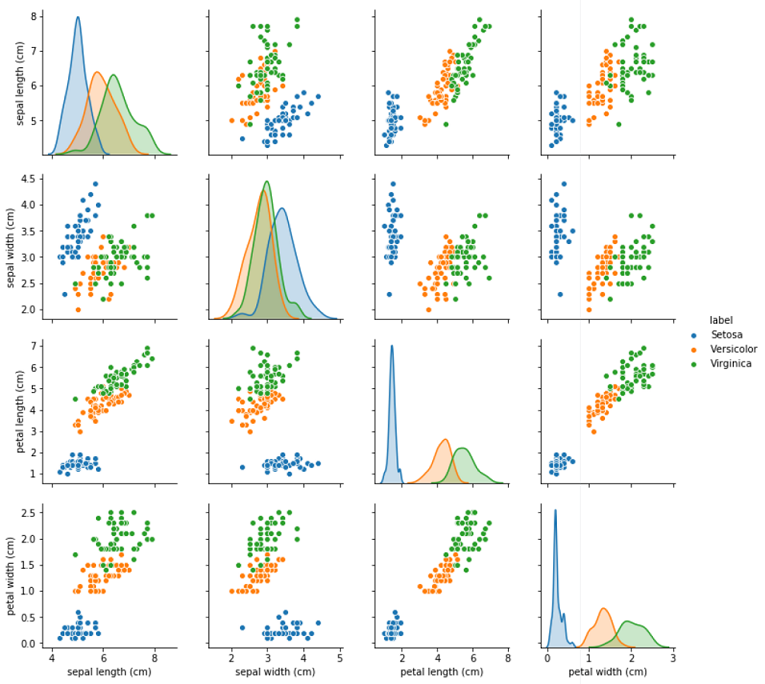Visualizing the Data

To better understand the types of data distributions that KNN would excel at classifying, we begin by generating some artificial data. The first function generates our data in such a manner that it perfectly separates the data based on the threshold specified. The second function alters the data generated from the first by introducing some randomness into the labels. Instead of perfect separation, we now have some probability, that we can set, whereby close by observations can now have the opposite label, thereby making our algorithm’s classification task much harder.

def gen_data(num_points):
# make empty arrays
x = np.zeros((num_points, 2))
y = np.zeros((num_points, 1))

# loop over length of arrays
for i in range(len(x)):
for j in range(2): # loop over number of features

x[i] = np.random.uniform(0, 1) # generate data between [0, 1]
x[i] = np.random.uniform(0, 1) # generate data between [0, 1]

# set arbitrary comparison to generate y labels
if x[i, 0] <= x[i, 1]:
y[i] = 0
else:
y[i] = 1

return ({'X': x, 'Y': y}) # return a dictionary

def mix_data(threshold1, threshold2):
for i in range(len(X)):
gen_random_value = np.random.random_sample()
if all([X[i, 0] >= threshold1, X[i, 1] >= threshold2]):
indx = i
if gen_random_value > .5:
Y[indx] = 1
else:
Y[indx] = 0

We generate data like so:

data = gen_data(600)
X = data['X'] # 600 observations; 2 features
Y = data['Y'] # 600 labels
Y = Y.ravel() # reshape (600,) for classifier use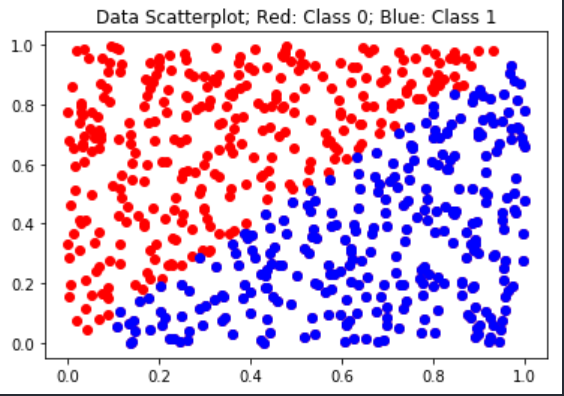Visualizing the Data

With our data generated, we can now create our own KNN classifier so that we can better understand what exactly sklearn is doing when we use its classifier. To create our own KNN classifier, we need to create the classifier and a function that calculates the Euclidean distance between points. We begin with Euclidean distance:

def EuclideanDistance(v1, v2):
# calculates the distance between two points
sum = 0.0
for index in range(len(v1)): # loop over length of container
sum += (v1[index] - v2[index]) ** 2 # square for positive
return sum ** 0.5 # square root to undo

Next, we construct a KNN (K=1) classifier that uses our Euclidean distance algorithm to determine the distances and labels between two points. In essence, the class calculates the distances between the features in the training and test data sets. Once the algorithm finds the lowest distance, it then finds the label at its appropriate index location and stores it in a results container.

class NN():
# initialize an instance of the class
def __init__(self, metric = EuclideanDistance):
self.metric = metric

# prepare the data
def nn_fit(self, train_data, train_labels):
self.train_data = train_data
self.train_labels = train_labels

# make a prediction
def nn_predict_item(self, item):
best_dist, best_label = 1.0e10, None
for i in range(len(self.train_data)):
dist = self.metric(self.train_data[i], item)
if dist < best_dist:
best_label = self.train_labels[i]
best_dist = dist
return best_label

# make predictions for each test example and return results.
def nn_predict(self, test_data):
results = []
for item in test_data:
results.append(self.nn_predict_item(item))
return results

We can now run our homemade classifier like so and view our results on our synthetic data:

# prepare data for classification
shuffle = np.random.permutation(np.arange(X.shape)) # shuffle data
X, Y = X[shuffle], Y[shuffle] # apply shuffle
train_data, train_labels = X[:400], Y[:400] # prepare train and test sets
test_data, test_labels = X[400::], Y[400::]

clf = NN() # instantiate the class
clf.nn_fit(train_data, train_labels) # store the data
preds = clf.nn_predict(test_data) # generate predictions from test data
wrong_labels = [] # storage container

correct, total = 0, 0 # generate counts
for pred, label in zip(preds, test_labels): # loop through consecutively
if pred == label: # if prediction matches the actual label, add 1
correct += 1
else: # otherwise, add 1 to the error
wrong_labels.append((pred, label))
total += 1

pt = PrettyTable() # create a nice table
pt.field_names = ["Total", "Correct", "Accuracy"]
print(pt)
## +-------+---------+----------+
## | Total | Correct | Accuracy |
## +-------+---------+----------+
## |  200  |   198   |   0.99   |
## +-------+---------+----------+

Unsurprisingly given the near perfect separation between data points, we can see that our algorithm does exceptionally well classifying the data. However, to illustrate the challenges associated with KNN for messy and overlapping data points using a distance metric, let’s alter the data using the mix data function above, yielding data that looks like:

data = gen_data(600)
X = data['X'] # 600 observations; 2 features
Y = data['Y'] # 600 labels
Y = Y.ravel() # reshape (600,) for classifier use
mix_data(0.0, 0.0)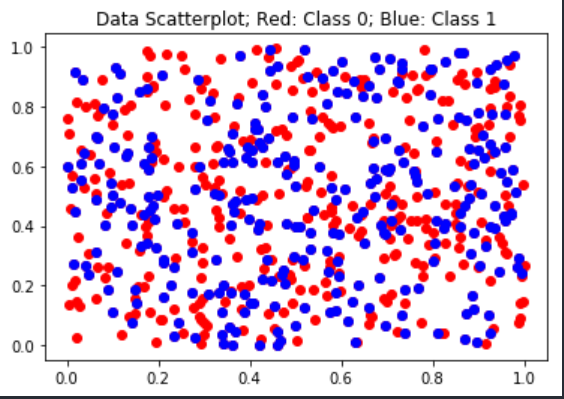Visualizing the Data

# prepare data for classification
shuffle = np.random.permutation(np.arange(X.shape)) # shuffle data
X, Y = X[shuffle], Y[shuffle] # apply shuffle
train_data, train_labels = X[:400], Y[:400] # prepare train and test sets
test_data, test_labels = X[400::], Y[400::]

clf = NN() # instantiate the class
clf.nn_fit(train_data, train_labels) # store the data
preds = clf.nn_predict(test_data) # generate predictions from test data
wrong_labels = [] # storage container

correct, total = 0, 0 # generate counts
for pred, label in zip(preds, test_labels): # loop through consecutively
if pred == label: # if prediction matches the actual label, add 1
correct += 1
else: # otherwise, add 1 to the error
wrong_labels.append((pred, label))
total += 1

pt = PrettyTable() # create a nice table
pt.field_names = ["Total", "Correct", "Accuracy"]
print(pt)
## +-------+---------+----------+
## | Total | Correct | Accuracy |
## +-------+---------+----------+
## |  200  |   105   |  0.525   |
## +-------+---------+----------+

With very messy or non-separated data, we can see that KNN struggles to make accurate predictions thereby providing support for the thesis of this demonstration. To see that our homemade classifier replicates sklearn’s predictions, we fit the same data to sklearn’s KNN class:

# load python
library(reticulate)
use_python('C:/Users/Andrew/Anaconda3/')
library(knitr)
model = KNeighborsClassifier(n_neighbors = 1) # instantiate KNN
model.fit(train_data, train_labels) # fit x, y

# generate results
## KNeighborsClassifier(algorithm='auto', leaf_size=30, metric='minkowski',
##                      metric_params=None, n_jobs=None, n_neighbors=1, p=2,
##                      weights='uniform')
predicted_y = model.predict(test_data) # predict labels from dev data
accuracy = accuracy_score(test_labels, predicted_y) # compare actual to predicted
print(accuracy)
## 0.525

With the intuition of KNN established, let’s see how KNN does on real data. MNIST (Modified National Institute of Standards and Technology database) is a famous data set used to formulate and test new algorithms among researchers and applied practitioners. In the chunk below, we begin by loading our data using sklearn’s handy package to access MNIST. We then rescale the X array between 0 and 1 by dividing by its maximum and then we shuffle the data and establish some training and test sets.

# load MNSIT from https://www.openml.org/d/554 via fetch_openml
X, Y = fetch_openml(name='mnist_784', return_X_y=True, cache=False)

# rescale grayscale values to [0,1].
X = X / 255.0

# shuffle the data
shuffle = np.random.permutation(np.arange(X.shape)) # generate unique random indices
X, Y = X[shuffle], Y[shuffle] # apply shuffle

# prepare some datasets
test_data, test_labels = X[61000:], Y[61000:]
dev_data, dev_labels = X[60000:61000], Y[60000:61000]
train_data, train_labels = X[:60000], Y[:60000]
mini_train_data, mini_train_labels = X[:1000], Y[:1000]

Since MNIST is a data set of handwritten digits, we can visualize the data thanks to imshow from matplotlib. In the chunk below, we collect 10 examples of all 10 digits and display them in a 2x2 matrix.

# 3 Visualize the Data

def visualize_mnist(label_array):
fig, ax = plt.subplots(10, 10, figsize = (10,10))  # create 10x10 subplots

for i in range(0, 10): # initiate loop
find_digit = np.where(label_array == str(i)) # find numbers 0-9
find_digit = mini_train_data[find_digit][0:10] # truncate data

for j in range(0, 10): # initiate loop
working_item = find_digit[j] # pull individual arrays
working_item.shape = (28, 28) # reshape for viewing
working_item = 255 - working_item # subtract itself for white background
ax[i, j].imshow(working_item, cmap = 'gray') # plot the image
ax[i, j].set_xticks([]) # remove axis ticks for clarity
ax[i, j].set_yticks([]) # remove axis ticks for clarity

return plt.show()

visualize_mnist(mini_train_labels)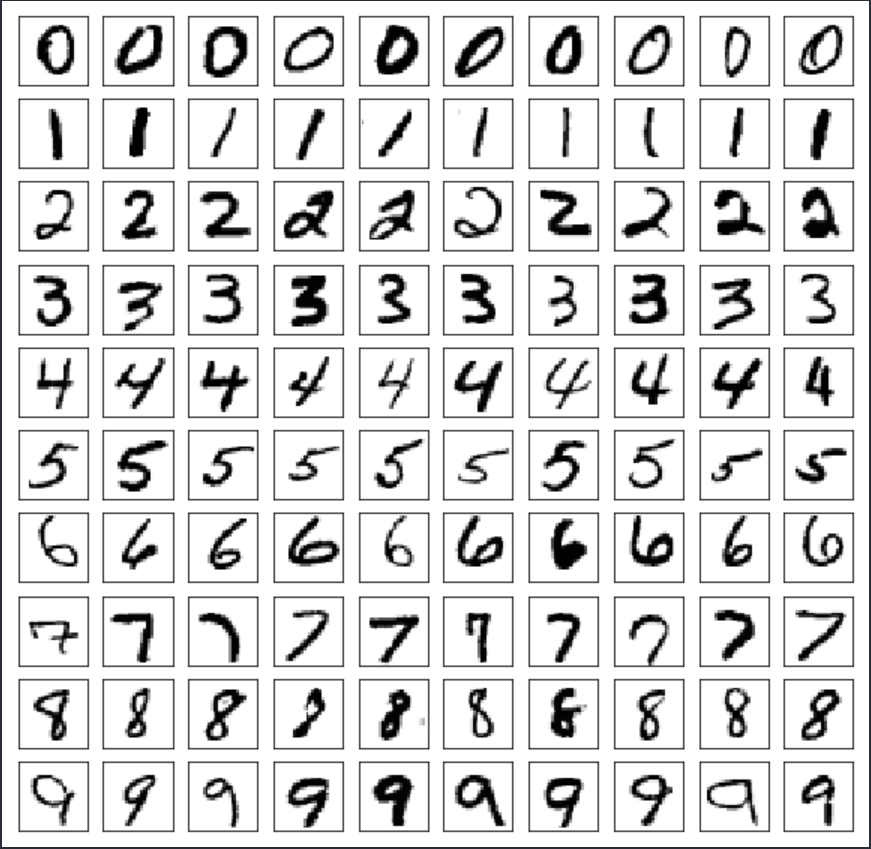Visualizing the Data

# 4 Running an Initial Model on MNIST

container = [[] for i in range(0, 2)] # create two storage containers
set_of_k = [1, 3, 5, 7, 9] # set number of k to loop through

for k in set_of_k: # begin loop
# instantiate model
model = KNeighborsClassifier(n_neighbors = k) # instantiate KNN
model.fit(mini_train_data, mini_train_labels) # fit x, y

# generate results
predicted_y = model.predict(dev_data) # predict labels from dev data
accuracy = accuracy_score(dev_labels, predicted_y) # compare actual to predicted

# store data for use later
container.append(k) # append K
container.append(accuracy) # append accuracy

if k == 1: # set if condition for k = 1 metric
print(classification_report(dev_labels, predicted_y)) # compare actual to predicted
## KNeighborsClassifier(algorithm='auto', leaf_size=30, metric='minkowski',
##                      metric_params=None, n_jobs=None, n_neighbors=1, p=2,
##                      weights='uniform')
##               precision    recall  f1-score   support
##
##            0       0.95      0.95      0.95       106
##            1       0.89      0.98      0.93       118
##            2       0.90      0.79      0.84       106
##            3       0.93      0.87      0.90        97
##            4       0.91      0.85      0.88        92
##            5       0.86      0.88      0.87        88
##            6       0.92      0.92      0.92       102
##            7       0.85      0.94      0.89       102
##            8       0.83      0.77      0.80        94
##            9       0.80      0.86      0.83        95
##
##     accuracy                           0.88      1000
##    macro avg       0.88      0.88      0.88      1000
## weighted avg       0.89      0.88      0.88      1000
##
## KNeighborsClassifier(algorithm='auto', leaf_size=30, metric='minkowski',
##                      metric_params=None, n_jobs=None, n_neighbors=3, p=2,
##                      weights='uniform')
## KNeighborsClassifier(algorithm='auto', leaf_size=30, metric='minkowski',
##                      metric_params=None, n_jobs=None, n_neighbors=5, p=2,
##                      weights='uniform')
## KNeighborsClassifier(algorithm='auto', leaf_size=30, metric='minkowski',
##                      metric_params=None, n_jobs=None, n_neighbors=7, p=2,
##                      weights='uniform')
## KNeighborsClassifier(algorithm='auto', leaf_size=30, metric='minkowski',
##                      metric_params=None, n_jobs=None, n_neighbors=9, p=2,
##                      weights='uniform')
pt = PrettyTable()
pt.field_names = ["k", "accuracy"]
for i in range(0, 5):
print(pt)
## +---+----------+
## | k | accuracy |
## +---+----------+
## | 1 |  0.884   |
## | 3 |  0.876   |
## | 5 |  0.882   |
## | 7 |  0.877   |
## | 9 |  0.875   |
## +---+----------+

There are a number of results worth interpreting here. First, we can see that the most difficult digit is 8; owing to its low f1-score, which is a weighted average of the recall and precision score. The recall score of 0.77 makes the problem of identifying 8 most clear because recall is the ratio of 8’s labelled correctly to those labelled correctly and those labelled incorrectly but should not have been, or: $$\frac{\text{True Positive}}{\text{True Positive + False Negative}}$$. Second, given our sample, we can see that as K increases, we gain little predictive power. However, as we will see, this is largely a function of our sample size where n = 1000.

# 5 MNIST: Increasing Sample Size

container = [[] for i in range(0, 3)] # create 3 containers for use later
train_sizes = [100, 200, 400, 800, 1600, 3200, 6400, 12800, 25600] # set training data sizes
k = 1 # set KNN to 1
accuracies = [] # store accuracies for use later

for size in train_sizes: # start loop
# instantiate the model and calculate the time
start_time = time.time() # start time calcs
model = KNeighborsClassifier(n_neighbors = k) # instantiate KNN
train_x = train_data[0:size] # set x training data sizes via loop
train_y = train_labels[0:size] # set y training data sizes via loop
model = KNeighborsClassifier(n_neighbors = k) # instantiate KNN
model.fit(train_x, train_y) # fit x, y

# generate results and stop time
predicted_y = model.predict(dev_data) # predict labels from dev data
accuracy = accuracy_score(dev_labels, predicted_y) # compare actual to predicted
end_time = time.time() # stop time
elapsed_time = end_time - start_time # calculate the time

# store data for use later
container.append(size) # append size
container.append(accuracy) # append accuracy
container.append(elapsed_time) # append time
accuracies.append(accuracy) # used later
## KNeighborsClassifier(algorithm='auto', leaf_size=30, metric='minkowski',
##                      metric_params=None, n_jobs=None, n_neighbors=1, p=2,
##                      weights='uniform')
## KNeighborsClassifier(algorithm='auto', leaf_size=30, metric='minkowski',
##                      metric_params=None, n_jobs=None, n_neighbors=1, p=2,
##                      weights='uniform')
## KNeighborsClassifier(algorithm='auto', leaf_size=30, metric='minkowski',
##                      metric_params=None, n_jobs=None, n_neighbors=1, p=2,
##                      weights='uniform')
## KNeighborsClassifier(algorithm='auto', leaf_size=30, metric='minkowski',
##                      metric_params=None, n_jobs=None, n_neighbors=1, p=2,
##                      weights='uniform')
## KNeighborsClassifier(algorithm='auto', leaf_size=30, metric='minkowski',
##                      metric_params=None, n_jobs=None, n_neighbors=1, p=2,
##                      weights='uniform')
## KNeighborsClassifier(algorithm='auto', leaf_size=30, metric='minkowski',
##                      metric_params=None, n_jobs=None, n_neighbors=1, p=2,
##                      weights='uniform')
## KNeighborsClassifier(algorithm='auto', leaf_size=30, metric='minkowski',
##                      metric_params=None, n_jobs=None, n_neighbors=1, p=2,
##                      weights='uniform')
## KNeighborsClassifier(algorithm='auto', leaf_size=30, metric='minkowski',
##                      metric_params=None, n_jobs=None, n_neighbors=1, p=2,
##                      weights='uniform')
## KNeighborsClassifier(algorithm='auto', leaf_size=30, metric='minkowski',
##                      metric_params=None, n_jobs=None, n_neighbors=1, p=2,
##                      weights='uniform')
pt = PrettyTable()
pt.field_names = ["Training Size", "Accuracy", "Time Elapsed"]
for i in range(0, 9):
print(pt)
## +---------------+----------+--------------+
## | Training Size | Accuracy | Time Elapsed |
## +---------------+----------+--------------+
## |      100      |  0.702   |     0.1      |
## |      200      |  0.791   |     0.18     |
## |      400      |  0.811   |     0.39     |
## |      800      |  0.866   |     0.7      |
## |      1600     |  0.905   |     1.46     |
## |      3200     |  0.927   |     3.1      |
## |      6400     |  0.939   |     6.46     |
## |     12800     |  0.952   |     13.6     |
## |     25600     |  0.963   |    29.19     |
## +---------------+----------+--------------+

We can see that the accuracy of our model, as shown by its accuracy score, increases as we increase the amount of data that it can learn from. Additionally, as the sample size doubles, computation time roughly doubles as well.

# 6 Confusion Matrix

k = 1 # set k = 1
model = KNeighborsClassifier(n_neighbors = k) # instantiate KNN
model.fit(mini_train_data, mini_train_labels) # x, y
## KNeighborsClassifier(algorithm='auto', leaf_size=30, metric='minkowski',
##                      metric_params=None, n_jobs=None, n_neighbors=1, p=2,
##                      weights='uniform')
predicted_y = model.predict(dev_data) # predict labels from dev data
accuracy = accuracy_score(dev_labels, predicted_y) # compare actual to predicted

# produce a confusion matrix
cm = confusion_matrix(dev_labels, predicted_y) # actual y vs predicted y
cm
## array([[101,   0,   1,   0,   0,   0,   1,   1,   2,   0],
##        [  0, 116,   1,   0,   0,   0,   0,   0,   1,   0],
##        [  1,   4,  84,   2,   2,   0,   2,   4,   6,   1],
##        [  0,   2,   0,  84,   0,   6,   0,   2,   3,   0],
##        [  0,   0,   1,   0,  78,   0,   0,   2,   0,  11],
##        [  2,   0,   0,   1,   1,  77,   5,   0,   2,   0],
##        [  1,   2,   1,   0,   1,   2,  94,   0,   1,   0],
##        [  0,   1,   1,   0,   0,   0,   0,  96,   0,   4],
##        [  1,   5,   4,   3,   1,   3,   0,   1,  72,   4],
##        [  0,   1,   0,   0,   3,   2,   0,   7,   0,  82]], dtype=int64)

The entry with “11” means the algorithm had the most confusion there. This means that the digits were actually “4”, but KNN predicted they were “9”. Let’s look at these examples in particular to see why it might be the case that KNN is getting confused here.

# find the errors:
fours = np.where(dev_labels == '4') # return index location of all 4s
predicted = np.where(predicted_y == '9') # return index location of all 9s
confused_indx = np.intersect1d(fours, predicted) # find the index location matches in both arrays

# plot the problematic 4s
fig, ax = plt.subplots(1, 11)  # create subplots
for i in range(len(confused_indx)):
indx = confused_indx[i]
img_arr = dev_data[indx]
img_arr.shape = (28, 28) # reshape for viewing
img_arr = 255 - img_arr # subtract itself for white background
ax[i].imshow(img_arr, cmap = 'gray') # plot the image
ax[i].set_xticks([]) # remove axis ticks for clarity
ax[i].set_yticks([]) # remove axis ticks for clarity

# find the errors:
nines = np.where(dev_labels == '9') # return index location of all 9s
predicted = np.where(predicted_y == '4') # return index location of all 4s
confused_indx = np.intersect1d(nines, predicted) # find the index location matches in both arrays

# plot the problematic 9s
fig, ax = plt.subplots(1, 3)  # create subplots
for i in range(len(confused_indx)):
indx = confused_indx[i]
img_arr = dev_data[indx]
img_arr.shape = (28, 28) # reshape for viewing
img_arr = 255 - img_arr # subtract itself for white background
ax[i].imshow(img_arr, cmap = 'gray') # plot the image
ax[i].set_xticks([]) # remove axis ticks for clarity
ax[i].set_yticks([]) # remove axis ticks for clarity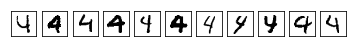Problematic 4s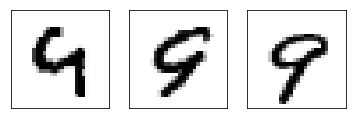Problematic 9s

The images above illustrate why that is the case nicely; they look remarkably similar in most cases. Identifying and pulling out specific errors is a great way to figure out how we might engineer solutions to these particular problems.

# 7 Feature Engineering: Image Smoothing

While our classifier does fairly well on our small training set with k=1, we can do much better by using an image processing technique called smoothing. This technique actually blurs the image by taking a weighted average of pixel values around each and every pixel and transforming the targeted pixel value to this new weighted average. Now, each pixel has far similar values and thus smaller distances between each other. We implement an image smoothing function below:

# blur function
def blur(training_data, blur_weight):
array = np.empty([0, 784])
container = []
current = np.copy(training_data) # copy required

for image in range(len(current)):
current_image = current[image] # pull current image
current_image = current_image.reshape(28, 28) # reshape

for row in range(len(current_image) - 1):
for column in range(len(current_image) - 1): # (row, column)

container.append(current_image[row - 1][column - 1]) # (-1, -1) pixel
container.append(current_image[row - 1][column]) # (-1, 0) pixel
container.append(current_image[row - 1][column + 1]) # (-1, 1) pixel
container.append(current_image[row][column - 1]) # (0, -1) pixel
container.append(current_image[row][column]) # (0, 0) pixel
container.append(current_image[row][column + 1]) # (0, 1) pixel
container.append(current_image[row + 1][column - 1]) # (1, -1) pixel
container.append(current_image[row + 1][column]) # (1, 0) pixel
container.append(current_image[row + 1][column + 1]) # (1, 1) pixel

current_image[row][column] = np.mean(container)*blur_weight # add weight to current pixel
container = [] # reset calculation container

array = np.append(array, [current_image], axis = 0) # add to array container

return array

We then create some new data by applying our blur function to our training data and in turn show case its results:

mini_train_blur = blur(training_data = mini_train_data, blur_weight = 0.6)
dev_train_blur = blur(training_data = dev_data, blur_weight = 0.6)
### show blur works
sample_img = mini_train_data
sample_img = sample_img.reshape(28, 28)
plt.imshow(sample_img)
plt.show()

sample_img2 = mini_train_blur
sample_img2 = sample_img2.reshape(28, 28)
plt.imshow(sample_img2)
plt.show()

###
sample_img3 = dev_data
sample_img3 = sample_img3.reshape(28, 28)
plt.imshow(sample_img3)
plt.show()

sample_img4 = dev_train_blur
sample_img4 = sample_img4.reshape(28, 28)
plt.imshow(sample_img4)
plt.show()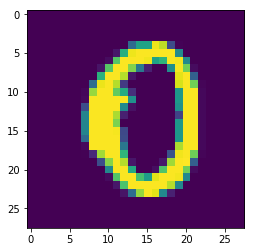Normal 0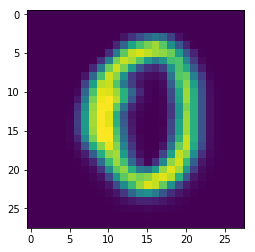Blurred 0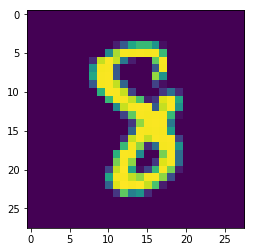Normal 8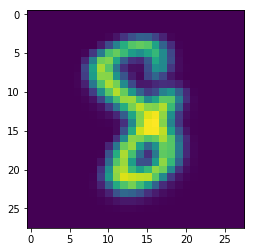Blurred 8

Next, we test to see how well our blurring function improves our predictions like so:

# Model 1: No Filter
model = KNeighborsClassifier(n_neighbors = 1) # instantiate KNN
fit = model.fit(mini_train_data, mini_train_labels) # x, y

predicted_y = fit.predict(dev_data) # predict labels from dev data
accuracy = accuracy_score(dev_labels, predicted_y) # compare actual to predicted
print('the accuracy of model 1 with no filter is', accuracy)

# Model 2: Gaussian Filtered Training Data
## the accuracy of model 1 with no filter is 0.884
model = KNeighborsClassifier(n_neighbors = 1) # instantiate KNN
fit = model.fit(mini_train_blur, mini_train_labels) # x, y

predicted_y = fit.predict(dev_data) # predict labels from dev data
accuracy = accuracy_score(dev_labels, predicted_y) # compare actual to predicted
print('the accuracy of model 2 filtered training data is', accuracy)

# Model 3: Gaussian Filtered Dev Data
## the accuracy of model 2 filtered training data is 0.864
model = KNeighborsClassifier(n_neighbors = 1) # instantiate KNN
fit = model.fit(mini_train_data, mini_train_labels) # x, y

predicted_y = fit.predict(dev_train_blur) # predict labels from dev data
accuracy = accuracy_score(dev_labels, predicted_y) # compare actual to predicted
print('the accuracy of model 3 filtered testing data is', accuracy)

# Model 4: Gaussian Filtered Training and Dev Data
## the accuracy of model 3 filtered testing data is 0.36
model = KNeighborsClassifier(n_neighbors = 1) # instantiate KNN
fit = model.fit(mini_train_blur, mini_train_labels) # x, y

predicted_y = fit.predict(dev_train_blur) # predict labels from dev data
accuracy = accuracy_score(dev_labels, predicted_y) # compare actual to predicted
print('the accuracy of model 4 filtered training and testing data is', accuracy)
## the accuracy of model 4 filtered training and testing data is 0.91

We can see that our feature engineering improves our accuracy by making it easier for KNN to classify the data.

# 8 Conclusion

This post walked through the application of the K Nearest Neighbors algorithm, demonstrating the conditions under which the algorithm excelled, did poorly, and could be improved through feature engineering.

# 9 Sources

• Matthias Feurer, Jan N. van Rijn, Arlind Kadra, Pieter Gijsbers, Neeratyoy Mallik, Sahithya Ravi, Andreas Mueller, Joaquin Vanschoren, Frank Hutter. OpenML-Python: an extensible Python API for OpenML. arXiv:1911.02490 [cs.LG], 2019

• LeCun, Yann. “The MNIST database of handwritten digits.” http://yann.lecun.com/exdb/mnist/ (1998).